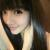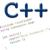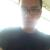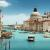# 从 C++ 到 Objective-C 的快速指南已翻译 100%

oschina 投递于 2014/05/10 07:37 (共 11 段, 翻译完成于 05-13)

### 背景

Objective C++是C++和Objective C的组合。你也能使用任何C++的东西混合到Objective C中，但是记住重新命名你的文件从.m到.mm

## 铛 - 铛!

```// Objective-C
- (int) foo : (int) a : (char) b {}
+ (int) foo : (int) a : (char) b {}

// C++
int foo(int a,char b) {}
static int foo(int a,char b) {}

// Objective-C
- (void) foo2  val1:(int) a; // named argument
// call
[obj foo2 val1:5];  // merely helper: You remember that 5 is assigned to param name val1.```

- 表示的是一个一般的成员函数（通过一个对象实体访问), 而 + 则表示一个静态成员函数, 不需要使用实体就能访问. 当然，像C++， 静态成员不能访问实体变量.

```// Objective-C
NSObject* ptr = ...; // some pointer
[ptr foo:5:3]; // call foo member with arguments 5 and 3
[NSObject staticfoo:5:3];  // call static function of NSOBject with arguments 4 and 3

// C++
CPPObject* ptr = ...; // some pointer
ptr->foo(5,3);
CPPObject::staticfoo(5,3);```

Objective-C 使用 [ ] 来调用成员函数并传入用：分割开的参数, 其对于ObjectiveC中ptr为nil的情况非常友好，在这种情况下“调用”将会被忽略掉（而在C++中，这种情况会抛出一个指针冲突异常 ). 这使得消除对nil对象的检查成为可能.

```// Objective-C
@protocol foo
- (void) somefunction;
@end

@interface c1 : NSObject<foo>

@end

@implementation c1
- (void) somefunction { ... }
@end

// C++
class foo
{
virtual void somefunction() = 0;
};

class c1 : public NSObject, public foo
{
void somefunction() { ... }
}```

```// Objective-C
NSObject* ptr = ...; // some pointer
[ptr somefunction:5:3]; // NSObject 不必一定为其编译而实现somefunction. 如果没有被实现的话，会引发异常.

// C++
CPPObject* ptr = ...; // some pointer
ptr->somefunction(5,3); // CPPObject 必须实现 somefunction() 否则程序根本就不会被编译.```

Objective-C 成员函数就是(Smalltalk中的) "消息"  而在Objective-C中时，我们则说接收者 (即指针) 会向一个选择器做出回应, 这意味着其实现了我们尝试去调用的虚函数. 当有一个接口是， C++ 对象必须实现其所有的成员函数. 而在 Objective-C 中这并不是必须的，因此我们可以向并不必须要实现它的某个地方发送一个”消息“  (如此就会引发一个异常).

```// Objective-C
NSObject* ptr = ...; // some pointer
if ([ptr respondsToSelector:@selector(somefunction::)]
[ptr somefunction:5:3];```

```// Objective-C
NSObject* ptr = ...; // some pointer
if ([ptr isKindOfClass:[foo class]]
[ptr somefunction:5:3];

// C++
CPPObject* ptr = ...; // some pointer
foo* f = dynamic_cast<foo*>(ptr);
if (f)
f->somefunction(5,3);```

```// Objective-C
NSObject* ptr = ...; // some pointer
if ([ptr conformsToProtocol:@protocol(foo)]
[ptr somefunction:5:3];

// C++
CPPObject* ptr = ...; // 某个也继承自foo的指针
foo* f = ptr; // 或者编译器会警告我们说ptr不能同foo兼容.
f->somefunction(5,3);```

void* 、 id 或者 SEL?

```// Objective-C
id ptr = ...; // some pointer
if ([ptr conformsToProtocol:@protocol(foo)]
[ptr somefunction:5:3];
SEL s = @selector(foo:); // a pointer to a function foo that takes 1 parameter

// C++
void* ptr = ...; // some pointer
foo* f = dynamic_cast<foo*>(ptr);
if (f)
f->somefunction(5,3);```

id 是通用的用于Objective-C类的类似于 void* 的东西. 你只能使用id而不是 void* 因为id可以通过ARC（稍后会详细介绍到它）编程一个可被管理的指针，而因此编译器需要在元指针类型和Objective-C指针之间做出区分. SEL 是一个用于选择器（C++函数指针）的通用类型，而你一般可以通过使用关键字@selector带上函数名字和:::::s的一个数字来创建一个选择器, 这取决于可以传入多少参数. 选择器实际上就是一个字符串，它会在运行时绑定到一个方法识别器.

```// Objective C
@class f2; // forward declaration
@interface f1 : NSOBject // Objective-C supports only public and single inheritance
{
int test; // default = protected
@public
int a;
int b;
f2* f;
}
- (void) foo;
@end

@implementation f1
- (void) foo
{
a = 5; // ok
self->a = 5; // ok
super.foo(); // parent call
}
@end

// C++
class f1 : public CPPObject
{
int test; // default = private
public:
class f2* f; // forward declaration
int a;
int b;
void foo();
}

void f1 :: foo()
{
a = 5; // ok
this->a = 5; // ok
CPPOBject::foo(); // parent call
}```

Objective-C中的实现范围在@implementation/@end 标记 (在 C++ 中我们可以将实现放在任何带有::范围操作符的地方)之中. 它使用@class关键字用于事先声明. Objective-C 默认带有 私有（private） 保护, 但仅用于数据成员(方法必须是公共的). Objective-C 使用 self 而不是 this ，并且它还可以通过super关键字调用它的父类.

```// Objective-C
NSObject* s = [NSObject alloc] init]; // can return nil if construction failed
[s retain]; // Increment the ref count

// C++
CPPObject* ptr = new CPPObject();   // can throw

// Objective-C
NSObject* s = [NSObject alloc] initwitharg:4];
[s release];

// C++
CPPOBject* ptr = new CPPOBject(4);
ptr->Release();```

Objective-C中的内存分配是通过静态成员方法alloc来实现的，所有 (做为NSObject后裔)的对象都有这个方法. self 在Objective-C中是可以被赋值的，而如果构建失败的话它就会设置成nil(而在C++中则会被抛出一个异常). 内存分配之后实际被构造器调用的是一个公共的成员函数，在Objective-C中默认的是init方法.

Objective-C 使用同COM益阳的引用计数方法, 而它也使用 retain 和 release (等同于IUnknown的 AddRef() 和 Release() 方法). 当引用计数到了0，则对象会从内存中被移除掉.

```// Objective C
@interface f1 : NSOBject // Objective-C supports only public and single inheritance
{
}
- (void) foo;
- (void) mt;

@end

@implementation f1

- (void) threadfunc : (NSInteger*) param
{
}

- (void) mt
{
}

- (void) foo
{
<div></div>}
@end```

Objective-C　有一些针对NSObject的内建功能，可以在另外一个线程中操作一个选择器 (== 调用一个成员), 在主线程中，等待一次调用等等 . 在NSObject 参见更多信息.

```// Objective-C
@interface f1 : NSObject
{
}
@property (weak) NSAnotherObject* f2; // 当没有其它强引用存在的时候，它将会被自动设置成.```

```@end

- (void) foo
{
NSObject* s = [NSObject alloc] init]; // 如果构造失败的话会返回nil
// use s
// end. Hooraah! Compiler will automatically call [s release] for us!
}```

Strings

```// Objective-C
NSString* s1 = @"hello";
NSString* s2 = [NSString stringWithUTF8String:"A C String"];
sprintf(buff,"%s hello to %@","there",s2);
const char* s3 = [s2 UTF8String];```

NSString 是一个Objective-C字符串的不可变表示. 你可以使用其一个静态方法，或者是一个带有@前缀的字符串文本来创建NSString. 你也可以使用 %@  来向printf家族函数来表示一个NSString,

```// Objective-C
NSArray* a1 = [NSArray alloc] initWithObjects: @"hello",@"there",nil];
NSString* first = [a1 objectAtIndex:0];```

NSArray和NSMutableArray是在Objective-C中处理数组的 两个类（两者的差异是，NSArray元素构造时必须通过构造函数，而NSMutableArray可以在之后更改）。典型构造函数的生效，你必须通过nil去作为“结尾元素”。排序/搜索/插入函数对于NSArray和NSMutableArray来说是一样的，在第一行中的例子它返回一个新的NSArray，而在NSMutableArray的例子里，它修改的是一个存在的对象。

```// C++
class MyString : public string
{
public:
void printmystring() { printf("%s",c_str()); }
};

// Objective-C
@interface MyString (NSString)
- (void) printmystring;
@end

@implementation MyString (NSString)
- (void) printmystring
{
printf("%@",self);
}
@end

// C++
MyString s1 = "hi";
s1.printmystring(); // ok
string s2 = "hello";
s2.printmystring(); // error, we must change s2 from string to MyString

// Objective-C
NSString* s2 = @"hello";
[s2 printmystring]; // valid. We extended NSString without changing types.```

C++依赖 继承机制来实现一个已知的类。这是很麻烦的，因为所有用户的实现类必须使用另外的类型名称（在例子中，MyString用来代替string）。Object-C通过使用 分类（Categories）允许扩展一个已知的类内 同型（same type 。上面链接中所有源代码在extension.h文件 （具有代表性的是像NSString+MyString.h这样的）中可以查看，上面例子中，我们立即就有可以调用新的成员函数，而不需要改变NSString类型为MyString。

```// Objective-C
// member function

// call

C++ 开发者使用 Objective-C 和 ARC 的重要提示

```// C++
class A
{
public:

NSObject* s;
A();
};

A :: A()
{
s = 0; // 可能会奔溃，这是常发生在发布模式下!
}```

```// C++
class A
{
public:

NSObject* s;
A();
};

A :: A() :s(0) // 现在编译器就知道确定 it&apos;s 是一次初始化了, 一次就不存在 [s release]
{
}```

```// Objective-C
NSObject* a = ...;
void* b = (__bridge void*)a; // 你必须在Objective-C和C类型支架使用 __bridge
void* c = (__bridge_retained void*)a; // 现在是一个+1的保留计数，而你必须在稍后释放这个对象
NSObject* d = (__bridge_transfer NSObject*)c; // 现在ARC取得了对象c的”拥有权“, 将其装换成一个ARC管理的NSObject.```

### 评论(30)#### 引用来自“四维海滨”的评论

+1
h#### 引用来自“leoking”的评论#### 引用来自“chape”的评论#### 引用来自“四维海滨”的评论